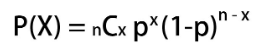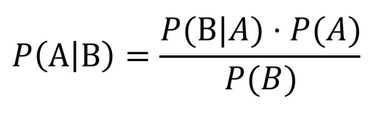##### Question

In: Statistics and Probability

# Assume we roll a fair four-sided die marked with 1, 2, 3 and 4. (a) Find...

Assume we roll a fair four-sided die marked with 1, 2, 3 and 4.
(a) Find the probability that the outcome 1 is first observed after 5 rolls.
(b) Find the expected number of rolls until outcomes 1 and 2 are both observed.
(c) Find the expected number of rolls until the outcome 3 is observed three times.
(d) Find the probability that the outcome 3 is observed exactly three times in 10 rolls
given that it is first observed after 5 rolls.
(e) Find the probability that the outcome 3 is first observed after 5 rolls given that
it is observed exactly three times in 10 rolls

## Solutions

##### Expert Solution

(a) probability that the outcome 1 is first observed after 5 rolls.

P(1) = 1/4

P(not 1) = 1-1/4 = 3/4

probability(outcome 1 is first observed after 5 rolls)

= P(1 doesn't come in 5 rolls)

= P(not 1)^5

= (3/4)^5

= 0.2373

(b) expected number of rolls until outcomes 1 and 2 are both observed.

The first number (either 1 or 2) will come up with probability 2/4 on each roll, and the number of rolls needed follows a geometric distribution, so 4/2 = 2 rolls are expected.

Then the remaining number comes up with probability 1/4, and similar to the first number, the reciprocal number of rolls are expected, or 4/1 = 4 rolls.

total rolls = 2+4 = 6

Thus 6 rolls are expected on average.

(c) expected number of rolls until the outcome 3 is observed three times.

expected no. of rolls for event to occur x times = x / (p(event))

P(3) = 1/4

expected no. of rolls for outcome 3 to occur 3 times = 3 / (1/4) = 12

expected no. of rolls for outcome 3 to occur 3 times = 12

(d) probability that the outcome 3 is observed exactly three times in 10 rolls given that it is first observed after 5 rolls.x=no. of 3

p=1/4

P(3 observed 3 times in 10 rolls | 3 not observed in first 5 rolls)

= P(3 observed 3 times in the 5 rolls afterr first 5 rolls)

= 5C3 * (1/4)^3 * (1 - 1/4)^(5-3)

= 0.0879

(e) probability that the outcome 3 is first observed after 5 rolls given that it is observed exactly three times in 10 rollsx=no. of 3

p=1/4

P(3 observed 3 times in 10 rolls) = 10C3 * (1/4)^3 * (1 - (1/4))^(10-3)

= 0.2503

P(3 is first observed after 5 rolls)

= P(3 doesn't come in 5 rolls)

= P(not 3)^5

= (1 - 1/4)^5

= 0.2373

P(3 observed 3 times in 10 rolls | 3 is first observed after 5 rolls)

= 0.0879 {from previous part}P( 3 is first observed after 5 rolls | 3 observed 3 times in 10 rolls)

= P(3 observed 3 times in 10 rolls | 3 is first observed after 5 rolls)*P(3 is first observed after 5 rolls) / P(3 observed 3 times in 10 rolls)

= 0.0879 * 0.2373 / 0.2503

= 0.0833

P( 3 is first observed after 5 rolls | 3 observed 3 times in 10 rolls) = 0.0833

## Related Solutions

##### Let X equal the outcome (1, 2 , 3 or 4) when a fair four-sided die...
Let X equal the outcome (1, 2 , 3 or 4) when a fair four-sided die is rolled; let Y equal the outcome (1, 2, 3, 4, 5 or 6) when a fair six-sided die is rolled. Let W=X+Y. a. What is the pdf of W? b What is E(W)?
##### Assume that you have a fair 6 sided die with values {1, 2, 3, 4, 5,...
Assume that you have a fair 6 sided die with values {1, 2, 3, 4, 5, 6} and a fair 12 sided die with values {1, 2, 3, 4, 5, 6, 7, 8, 9, 10, 11, 12}. A discrete random variable is generated by rolling the two dice and adding the numerical results together. (a) Create a probability mass function that captures the probability of all possible values of this random variable. You may use R or draw the pmf...
##### Consider rolling both a fair four-sided die numbered 1-4 and a fair six-sided die numbered 1-6...
Consider rolling both a fair four-sided die numbered 1-4 and a fair six-sided die numbered 1-6 together. After rolling both dice, let X denote the number appearing on the foursided die and Y the number appearing on the six-sided die. Define W = X +Y . Assume X and Y are independent. (a) Find the moment generating function for W. (b) Use the moment generating function technique to find the expectation. (c) Use the moment generating function technique to find...
##### Consider a fair four-sided die numbered 1-4 and a fair six-sided die numbered 1-6, where X...
Consider a fair four-sided die numbered 1-4 and a fair six-sided die numbered 1-6, where X is the number appearing on the four-sided die and Y is the number appearing on the six-sided die. Define W=X+Y when they are rolled together. Assuming X and Y are independent, (a) find the moment generating function for W, (b) the expectation E(W), (c) and the variance Var(W). Use the moment generating function technique to find the expectation and variance.
##### 17#13 Suppose we roll a fair six-sided die and sum the values obtained on each roll,...
17#13 Suppose we roll a fair six-sided die and sum the values obtained on each roll, stopping once our sum exceeds 289. Approximate the probability that at least 76 rolls are needed to get this sum.
##### Suppose you are rolling a fair four-sided die and a fair six-sided die and you are...
Suppose you are rolling a fair four-sided die and a fair six-sided die and you are counting the number of ones that come up. a) Distinguish between the outcomes and events. b) What is the probability that both die roll ones? c) What is the probability that exactly one die rolls a one? d) What is the probability that neither die rolls a one? e) What is the expected number of ones? f) If you did this 1000 times, approximately...
##### Suppose a 9-sided and a 4-sided die are rolled. Find these probabilities. a. P(roll sum of...
Suppose a 9-sided and a 4-sided die are rolled. Find these probabilities. a. P(roll sum of less than 5 or roll doubles) b. P(roll a sum greater than 6 for the first time on the sixth roll)
##### Suppose we roll a fair 6 sided die with the numbers [1,6] written on them. After...
Suppose we roll a fair 6 sided die with the numbers [1,6] written on them. After the first die roll we roll the die ? times where ? is the number on the first die roll. The number of points you score is the sum of the face-values on all die rolls (including the first). What is the expected number of points you will score?
##### Find the conditional​ probability, in a single roll of two fair​ 6-sided dice, that neither die...
Find the conditional​ probability, in a single roll of two fair​ 6-sided dice, that neither die is a three​,given that the sum is greater than 7.
##### Assume that a fair die is rolled. The sample space is , 1, 2, 3, 4,...
Assume that a fair die is rolled. The sample space is , 1, 2, 3, 4, 56 , and all the outcomes are equally likely. Find P Greater than 4 . Write your answer as a fraction or whole number. Assume that a fair die is rolled. The sample space is , 1, 2, 3, 4, 56 , and all the outcomes are equally likely. Find P Greater than 4 . Write your answer as a fraction or whole number.